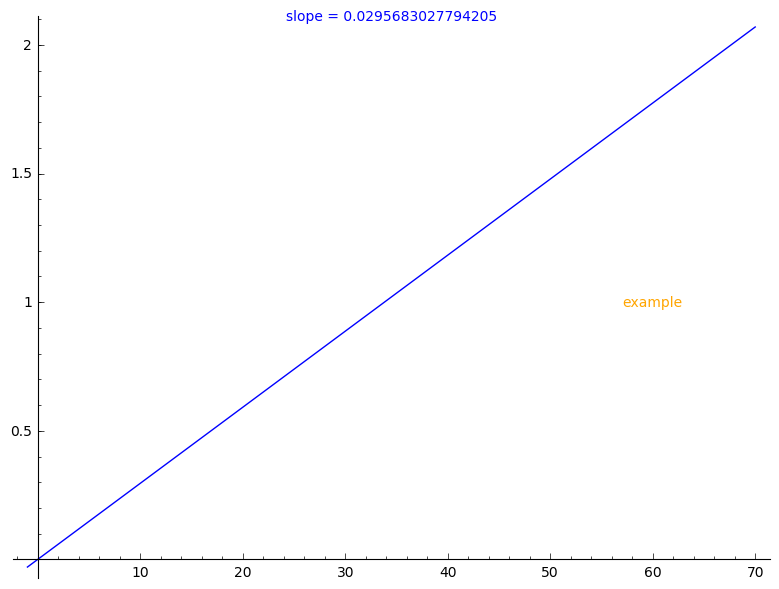# Putting the results of a function as text into a plot

How can I put the results of functions into a plot? I can't find any examples.

f(x) = x/33.82
plotEquation = plot(f(x), -1, 70)
equationDifferential = f.diff()(x)
big_image = plotEquation
# big_image = plotEquation + SOME CODE THAT PUTS THE RESULT OF f.diff()(x) in the plot.
big_image.show()

edit retag close merge delete

Sort by » oldest newest most voted

You may use text to create a text 2D graphics object and + to join the graphics:

sage: f(x)=x/33.82
sage: plotEquation = plot(f(x),-1,70)
sage: equationDifferential = f.diff()(x)
sage: big_image = plotEquation
sage: equationDifferential
0.0295683027794205
sage: t = text("slope = {}".format(equationDifferential), (.5, 1), axis_coords=True)
sage: s = text("example", (60, 1), color='orange')
sage: big_image = plotEquation + t + s
sage: big_imageNote that axis_coords=True changes the coordinate system as described by the documentation of text:

   * "axis_coords" - (default: False) If True, use axis coordinates,
so that (0,0) is the lower left and (1,1) upper right, regardless
of the x and y range of plotted values.

more

@SteveF - if this solves your question, please accept this answer by clicking the "accept" icon (tick mark) at the top left of the answer. This will mark the answer as accepted and your question as solved.

In complement to @Sébastien answer, note that you can include mathematical expressions in the displayed text by transforming them into a LaTeX string first: if f(x) is a symbolic function that you have defined, you can do

text("My function: " + "$" + latex(f(x)) + "$", (60, 1))